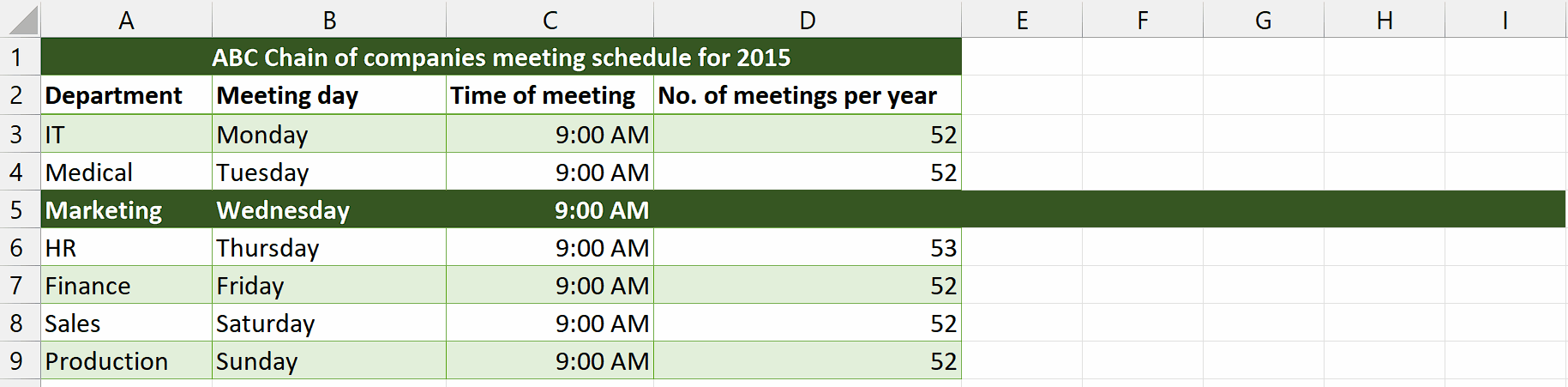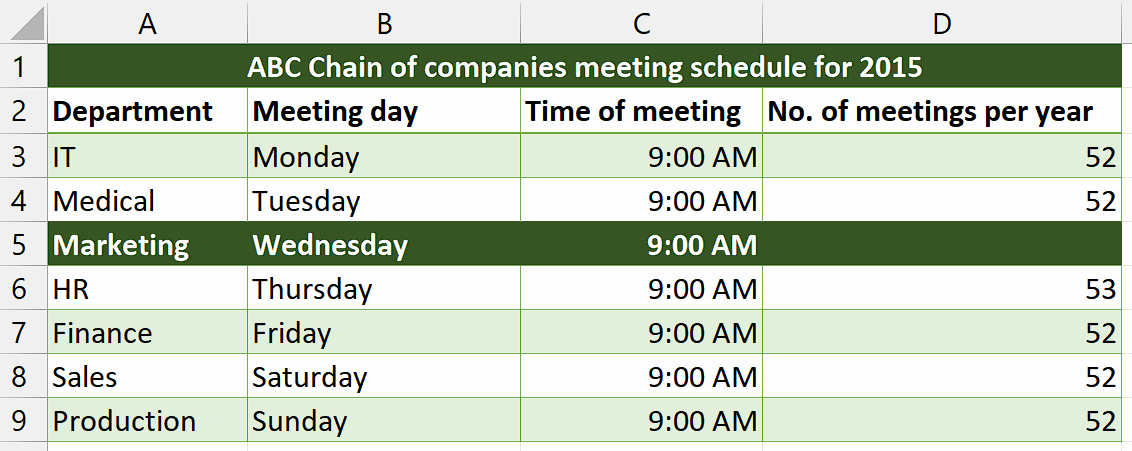# How to calculate Wednesdays in 2015

The provided dataset presents the meeting schedule for the ABC Chain of companies throughout the year 2015. The dataset includes information about the various departments within the company, their corresponding meeting days, the time of each meeting, and the number of meetings conducted per year. By considering that the Marketing Department conducts its meetings on Wednesdays, we will calculate the total number of meetings that took place in the department during the year 2015, which corresponds to the total number of Wednesdays in that year.

## Understanding Functions and their syntax

SUMPRODUCT Function:

The SUMPRODUCT function is a mathematical function commonly used in spreadsheet applications like Microsoft Excel or Google Sheets. It allows you to multiply corresponding elements in multiple arrays or ranges and then sum the products of those multiplications. The result is a single value that represents the sum of the products.

The syntax for the SUMPRODUCT function is as follows:

=SUMPRODUCT(array1, array2, …)

Here’s a breakdown of the syntax elements:

array1, array2, … –  These are the arrays or ranges you want to multiply and sum. You can provide multiple arrays separated by commas. Each array can be a range of cells, or you can explicitly define an array using curly braces or other array functions. The arrays should have the same dimensions or be single rows or columns.

WEEKDAY Function:

The WEEKDAY function in Excel is used to determine the day of the week for a given date. It returns a number representing the day of the week, where Sunday is considered as 1 and Saturday is considered as 7.

The syntax of the WEEKDAY function is as follows:

=WEEKDAY(serial_number, [return_type])

Here is the breakdown of the syntax:

The serial_number argument is the date for which you want to find the day of the week. It can be entered directly as a date enclosed in quotation marks (e.g., “6/15/2023”), or it can be a cell reference to a cell containing a date.

The return_type argument is optional and determines the type of return value you want from the function. It can take the following values:

1 or omitted: Returns numbers from 1 (Sunday) to 7 (Saturday).

2: Returns numbers from 1 (Monday) to 7 (Sunday).

3: Returns numbers from 0 (Monday) to 6 (Sunday).

11: Returns numbers from 1 (Monday) to 7 (Sunday), with Monday as the first day of the week.

DATE Function:

The DATE function in Excel is used to create a date by specifying the year, month, and day. It returns the corresponding serial number that represents the date.

The syntax of the DATE function is as follows:

=DATE(year, month, day)

The breakdown of the syntax of this function is as follows:

year: A required argument that specifies the year of the date. It can be an integer between 1900 and 9999 or a cell reference containing a valid year.

month: A required argument that specifies the month of the date. It can be an integer between 1 and 12 or a cell reference containing a valid month.

day: A required argument that specifies the day of the date. It can be an integer between 1 and 31 or a cell reference containing a valid day.

The DATE function will return a date value corresponding to the provided year, month, and day. This date value is a serial number representing the date in Excel’s internal date format.

ROW Function:

The ROW function in Excel is a built-in function that returns the row number of a cell reference. It can be used to retrieve the row number of a specific cell or to generate a series of numbers representing the rows in a range.

The syntax of the ROW function is as follows:

=ROW([reference])

The reference parameter is optional. It specifies the cell reference for which you want to obtain the row number. If the reference parameter is omitted, the function will return the row number of the cell in which the formula is entered.

INDIRECT Function:

The INDIRECT function in Excel is a powerful tool that allows you to indirectly reference a cell or range of cells in a formula. It takes a text string as input and converts it into a valid cell reference, which can then be used in a formula to retrieve the value or perform calculations.

The syntax of the INDIRECT function is as follows:

=INDIRECT(ref_text, [a1])

The breakdown of the function’s syntax is as follows:

ref_text: This is a required argument and represents the text string that specifies the cell reference you want to indirectly reference. It can be a cell reference (e.g., A1) or a named range (e.g., “Sales_Total”).

a1 (optional): This argument is a logical value that specifies the type of cell reference to use. If a1 is TRUE or omitted, the function uses the A1 reference style, where columns are represented by letters and rows by numbers (e.g., A1, B2, C3). If a1 is FALSE, the function uses the R1C1 reference style, where both the rows and columns are represented by numbers (e.g., R1C1, R2C3).Wednesdays in a particular year, such as 2015, may be useful for various reasons such as knowing the number of Wednesdays in a year can help in scheduling events, meetings, or appointments that recur on Wednesdays. It allows for better organization and time management.

### Step 1 – Select the cell– First of all, select a cell in which you want to calculate the total number of Wednesdays in the year 2015.
– We will implement the formula in this cell to calculate our desired result.

### Step 2 – Write and implement the formula– After selecting the desired cell, write the following formula in the cell
=SUMPRODUCT(–(WEEKDAY(DATE(2015,1,ROW(INDIRECT(“1:365”))))=4))
– Then, press Enter and you’ll get your answer which is 52.

## Explanation of formula used in Step 2:

Let’s break down each component of the formula:

=SUMPRODUCT(–(WEEKDAY(DATE(2015,1,ROW(INDIRECT(“1:365”))))=4))

DATE(2015, 1, ROW(INDIRECT(“1:365”))): This part generates an array of dates from January 1, 2015, to December 31, 2015. The ROW(INDIRECT(“1:365”)) creates an array of numbers from 1 to 365, which represents the days of the year.

WEEKDAY(DATE(2015, 1, ROW(INDIRECT(“1:365”)))): The WEEKDAY function takes each date in the array generated in step 1 and returns a number representing the day of the week. By default, the function considers Sunday as 1 and Saturday as 7.

(WEEKDAY(DATE(2015, 1, ROW(INDIRECT(“1:365”)))) = 4): This expression compares the result of the WEEKDAY function to the value 4, which represents Wednesday. It returns an array of Boolean values where TRUE indicates that the corresponding date is a Wednesday, and FALSE indicates it is not.

–(WEEKDAY(DATE(2015, 1, ROW(INDIRECT(“1:365”)))) = 4): The double unary operator (–) converts the Boolean array into an array of 1s and 0s. TRUE is converted to 1, and FALSE is converted to 0.
SUMPRODUCT(–(WEEKDAY(DATE(2015, 1, ROW(INDIRECT(“1:365”)))) = 4)): The SUMPRODUCT function calculates the sum of the values in the array obtained in step 4. Since the array only contains 1s and 0s, the sum represents the count of Wednesdays in the year 2015.